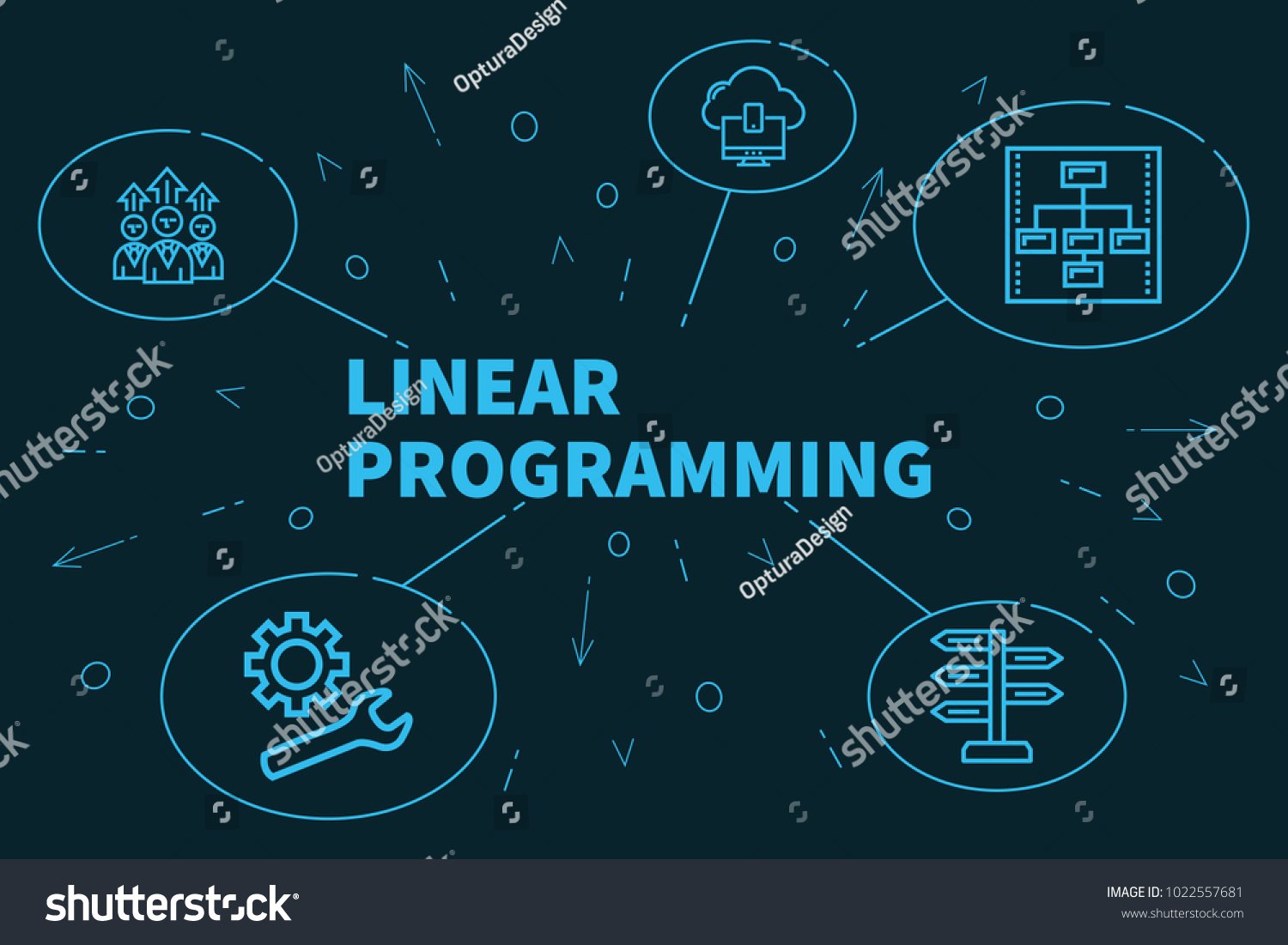The concept of linear programing sounds like an intense or complicated term right?. This was the impression I had till I came in contact with it as a topic in data analytics. The concept of linear programing is making good use of resources available to achieve goals and objectives.

It is a mathematical technique for maximizing or minimizing a linear function of several variables, such as output or cost. It is a method to achieve the best outcome in a mathematical model where the requirements are represented in linear relationships. The key feature of linear programing is resource optimization. The constraint in linear programing is the limited resources.

All linear programing problems must have the following five characteristics:

1. Objective function: Ina a problem, the objective function should be mentioned in a quantitative way.
2. Constraint: The most likely constraint is maximizing profit subject to limited resources. However, there might be some slight disparity in some situations. The limitation should be put in the mathematical way, regarding the given resources.
3. Non negativity: There are no negative value. You can only have positive numbers or zero.
4. Linearity: This linearity shows the consistency in the input and output. For example if you put 1 input, you are expected to get one output. The relationship between two or more variables in the function should be linear.
5. Finiteness : There always should be finite and infinite input and output numbers. If the function has infinite factors, the optimal solution will not be feasible.

Assumptions of Linear Programing

1. Conditions of Certainty: It means that numbers in the objectives and constraints are known with certainty and do change during the period being studied.
2. Resources are limited
3. There is only one objective.
4. The objective function is to be optimized. That is profit maximization or cost maximization always occurs at a corner point of the set of the feasible solution.
5. The relationship between objective function and constraints are linear.
6. There are a number of constraint or restrictions expressible in quantitative terms.
7. Data are available to specify the problem.
8. Non negativity constraint: In linear programing problems, we assume that all answers or variables are non negative. Negative values of physical quantities are an impossible situation. Anything that is a constraint must be measured.
9. Additively : This means that total of all activities quails the sum of each individual activity. In order words there is no interaction among all the activities of the resources. Data must be available to specify the problem
##### Written by Chika Laju-okorodudu
I am a Lawyer with a working experience in Human Resource Management. I love to explore and try new things...## My BLOGGING EXPERIENCE AS AN AID TO BETTER MY WRITING SKILL

in## EXAMS

in